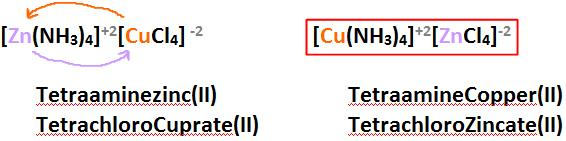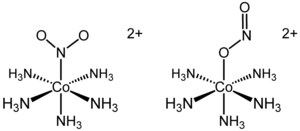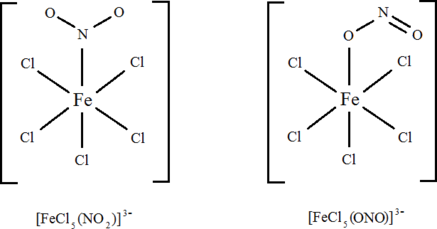# 2.4: Isomerism

Learning Objectives

• Explain the differences of Structural and Geometric isomerization in a coordination complexes or complex ions.
• Define Ionization, Linkage, and Coordination Isomerization (structural isomer classes)
• Define cis/tran and mer/fac isomerization (geometric isomer classes)

The existence of coordination compounds with the same formula but different arrangements of the ligands was crucial in the development of coordination chemistry. Two or more compounds with the same formula but different arrangements of the atoms are called isomers. Because isomers usually have different physical and chemical properties, it is important to know which isomer we are dealing with if more than one isomer is possible. As we will see, coordination compounds exhibit the same types of isomers as organic compounds, as well as several kinds of isomers that are unique. Isomers are compounds with the same molecular formula but different structural formulas and do not necessarily share similar properties. There are many different classes of isomers, like stereoisomers, enantiomers, and geometrical isomers. There are two main forms of isomerism: structural isomerism and stereoisomerism (spatial isomerism).

## Class I: Structural Isomers

Isomers that contain the same number of atoms of each kind but differ in which atoms are bonded to one another are called structural isomers, which differ in structure or bond type. For inorganic complexes, there are three types of structural isomers: ionization, coordination and linkage. Structural isomers, as their name implies, differ in their structure or bonding, which are separate from stereoisomers that differ in the spatial arrangement of the ligands are attached, but still have the bonding properties. The different chemical formulas in structural isomers are caused either by a difference in what ligands are bonded to the central atoms or how the individual ligands are bonded to the central atoms. When determining a structural isomer, you look at (1) the ligands that are bonded to the central metal and (2) which atom of the ligands attach to the central metal.

#### Structural Isomers: Ionization Isomerism

Ionization isomers occur when a ligand that is bound to the metal center exchanges places with an anion or neutral molecule that was originally outside the coordination complex. The geometry of the central metal ion and the identity of other ligands are identical. For example, an octahedral isomer will have five ligands that are identical, but the sixth will differ. The non-matching ligand in one compound will be outside of the coordination sphere of the other compound. Because the anion or molecule outside the coordination sphere is different, the chemical properties of these isomers is different. A hydrate isomer is a specific kind of ionization isomer where a water molecule is one of the molecules that exchanges places.

The difference between the ionization isomers can be view within the context of the ions generated when each are dissolved in solution. For example, when pentaamminebromocobalt(II) chloride is dissolved in water, $$\ce{Cl^{-}}$$ ions are generated:

$\ce{CoBr(NH_3)_5Cl {(s)} \rightarrow CoBr(NH_3)^{+}5 (aq) + Cl^{-} (aq)}$

whereas when pentaamminechlorocobalt(II) bromide is dissolved, $$\ce{Br^{-}}$$ ions are generated:

$\ce{CoCl(NH_3)_5Br {(s)} \rightarrow CoCl(NH_3)^{+}_{5} (aq) + Br^{-} (aq)}$

Notice that both anions are necessary to balance the charge of the complex, and that they differ in that one ion is directly attached to the central metal, but the other is not.

Solvate and Hydrate Isomerization: A special Type of Ionization Isomers

A very similar type of isomerism results from replacement of a coordinated group by a solvent molecule (Solvate Isomerism), which in the case of water is called Hydrate Isomerism. The best known example of this occurs for chromium chloride ($$\ce{CrCl_3 \cdot 6H_2O}$$) which may contain 4, 5, or 6 coordinated water molecules (assuming a coordination number of 6). The dot here is used essentially as an expression of ignorance to indicate that, though the parts of the molecule separated by the dot are bonded to one another in some fashion, the exact structural details of that interaction are not fully expressed in the resulting formula. Using Alfred Werner’s coordination theory that indicates that several of the water molecules are actually bonded directly (via coordinate covalent bonds) to the central chromium ion. In fact, there are several possible compounds that use the brackets to signify bonding in the complex and the the dots to signify "water molecules that are not bound to the central metal, but are part of the lattice:

• $$\ce{[CrCl_2(H_2O)_4]Cl \cdot 2H_2O}$$: bright-green colored
• $$\ce{[CrCl(H_2O)_5]Cl_2 \cdot H_2O}$$: grey-green colored
• $$\ce{[Cr(H_2O)_6]Cl_3}$$: violet colored

These isomers have very different chemical properties and on reaction with $$\ce{AgNO_3}$$ to test for $$\ce{Cl^{-}}$$ ions, would find 1, 2, and 3 $$\ce{Cl^{-}}$$ ions in solution, respectively.

Upon crystallization from water, many compounds incorporate water molecules in their crystalline frameworks. These "waters of crystallization" refers to water that is found in the crystalline framework of a metal complex or a salt, which is not directly bonded to the metal cation. In the first two hydrate isomers, there are water molecules that are artifacts of the crystallization and occur inside crystals. These water of crystallization is the total weight of water in a substance at a given temperature and is mostly present in a definite (stoichiometric) ratio.The $$\ce{[Cr(H_2O)_6]Cl_3}$$ hydrate isomer (left) is violet colored and the $$\ce{[CrCl(H_2O)_5]Cl_2 \cdot H_2O}$$ hydrate isomer is green-grey colored.

What are "Waters of Crystallization"?

A compound with associated water of crystallization is known as a hydrate. The structure of hydrates can be quite elaborate, because of the existence of hydrogen bonds that define polymeric structures. For example, consider the aquo complex $$\ce{NiCl_2 \cdot 6H_2O}$$ that consists of separated trans-[NiCl2(H2O)4] molecules linked more weakly to adjacent water molecules. Only four of the six water molecules in the formula are bound to the nickel (II) cation, and the remaining two are waters of crystallization as the crystal structure resolves.Structure of $$\ce{NiCl_2 \cdot 6H_2O}$$ salt with chlorine atoms (green), water molecules (red), and Ni metals (blue) indicated. (CC BY-SA 4.0; Smokefoot).

Water is particularly common solvent to be found in crystals because it is small and polar. But all solvents can be found in some host crystals. Water is noteworthy because it is reactive, whereas other solvents such as benzene are considered to be chemically innocuous.

### Structural Isomers: Coordination Isomerism

Coordination isomerism occurs compounds containing complex anionic and cationic parts can be thought of as occurring by interchange of some ligands from the cationic part to the anionic part. Hence, there are two complex compounds bound together, one with a negative charge and the other with a positive charge. In coordination isomers, the anion and cation complexes of a coordination compound exchange one or more ligands. For example, the $$\ce{[Co(NH3)6] [Cr(C2O4)3]}$$ compounds and $$\ce{[Co(C2O4)3] [Cr(NH3)6]}$$ compound are coordination isomers.Example $$\PageIndex{2}$$: Coordination IsomerizationLinkage isomerism occurs with ambidentate ligands that are capable of coordinating in more than one way. The best known cases involve the monodentate ligands: $$\ce{SCN^{-} / NCS^{-}}$$ and $$\ce{NO_2^{-} / ONO^{-}}$$. The only difference is what atoms the molecular ligands bind to the central ion. The ligand(s) must have more than one donor atom, but bind to ion in only one place. For example, the ($$\ce{NO2^{-}}$$) ion is a ligand can bind to the central atom through the nitrogen or the oxygen atom, but cannot bind to the central atom with both oxygen and nitrogen at once, in which case it would be called a polydentate rather than an ambidentate ligand.Figure $$\PageIndex{2}$$: Linkage Isomerism in the $$NO_2$$ ligand. This occurs when a particular ligand is capable of coordinating to a metal in two different and distinct ways. from Angel C. de Dios.

As with all structural isomers, the formula of the complex is unchanged for each isomer, but the properties may differ. The names used to specify the changed ligands are changed as well. For example, the $$\ce{NO2^{-}}$$ ion is called nitro when it binds with the $$\ce{N}$$ atom and is called nitrito when it binds with the $$\ce{O}$$ atom.

Example $$\PageIndex{3}$$: Linkage Isomerization

The cationic cobalt complex [Co(NH3)5(NO2)]Cl2 exists in two separable linkage isomers of the complex ion: (NH3)5(NO2)]2+.(left) The nitro isomer (Co-NO2) and (right) the nitrito isomer (Co-ONO)

When donation is from nitrogen to a metal center, the complex is known as a nitro- complex and when donation is from one oxygen to a metal center, the complex is known as a nitrito- complex. An alternative formula structure to emphasize the different coordinate covalent bond for the two isomers

• $$\ce{[Co(ONO)(NH_3)_5]Cl}$$: the nitrito isomer -O attached
• $$\ce{[Co(NO_2)(NH_3)_5]Cl}$$: the nitro isomer - N attached.

Another example of an ambidentate ligans is thiocyanate, SCN, which can attach at either the sulfur atom or the nitrogen atom. Such compounds give rise to linkage isomerism. Polyfunctional ligands can bond to a metal center through different ligand atoms to form various isomers. Other ligands that give rise to linkage isomers include selenocyanate, $$\ce{SeCN^{−}}$$ – isoselenocyanate, $$\ce{NCSe^{−}}$$ and sulfite, $$\ce{SO3^{2−}}$$.

Exercise $$\PageIndex{3}$$

Are $$\ce{[FeCl5(NO2)]^{3–}}$$ and $$\ce{[FeCl5(ONO)]^{3–}}$$ complex ions linkage isomers of each other?

Here, the difference is in how the ligand bonds to the metal. In the first isomer, the ligand bonds to the metal through an electron pair on the nitrogen. In the second isomer, the ligand bonds to the metal through an electron pair on one of the oxygen atoms. It's easier to see it:## Class 2: Geometric Isomers

The existence of coordination compounds with the same formula but different arrangements of the ligands was crucial in the development of coordination chemistry. Two or more compounds with the same formula but different arrangements of the atoms are called isomers. Because isomers usually have different physical and chemical properties, it is important to know which isomer we are dealing with if more than one isomer is possible. Recall that in many cases more than one structure is possible for organic compounds with the same molecular formula; examples discussed previously include n-butane versus isobutane and cis-2-butene versus trans-2-butene. As we will see, coordination compounds exhibit the same types of isomers as organic compounds, as well as several kinds of isomers that are unique.

### Geometric Isomers: Planar Isomers

Metal complexes that differ only in which ligands are adjacent to one another (cis) or directly across from one another (trans) in the coordination sphere of the metal are called geometrical isomers. They are most important for square planar and octahedral complexes.

Because all vertices of a square are equivalent, it does not matter which vertex is occupied by the ligand B in a square planar MA3B complex; hence only a single geometrical isomer is possible in this case (and in the analogous MAB3 case). All four structures shown here are chemically identical because they can be superimposed simply by rotating the complex in space:For an MA2B2 complex, there are two possible isomers: either the A ligands can be adjacent to one another (cis), in which case the B ligands must also be cis, or the A ligands can be across from one another (trans), in which case the B ligands must also be trans. Even though it is possible to draw the cis isomer in four different ways and the trans isomer in two different ways, all members of each set are chemically equivalent:Because there is no way to convert the cis structure to the trans by rotating or flipping the molecule in space, they are fundamentally different arrangements of atoms in space. Probably the best-known examples of cis and trans isomers of an MA2B2 square planar complex are cis-Pt(NH3)2Cl2, also known as cisplatin, and trans-Pt(NH3)2Cl2, which is actually toxic rather than therapeutic.The anticancer drug cisplatin and its inactive trans isomer. Cisplatin is especially effective against tumors of the reproductive organs, which primarily affect individuals in their 20s and were notoriously difficult to cure. For example, after being diagnosed with metastasized testicular cancer in 1991 and given only a 50% chance of survival, Lance Armstrong was cured by treatment with cisplatin.

Square planar complexes that contain symmetrical bidentate ligands, such as [Pt(en)2]2+, have only one possible structure, in which curved lines linking the two N atoms indicate the ethylenediamine ligands:Exercise $$\PageIndex{4}$$

Draw the cis and trans isomers of the following compounds:

1. (NH3)2IrCl(CO)
2. (H3P)2PtHBr
3. (AsH3)2PtH(CO)

Exercise $$\PageIndex{5}$$

Only one isomer of (tmeda)PtCl2 is possible [tmeda = (CH3)2NCH2CH2N(CH3)2; both nitrogens connect to the platinum]. Draw this isomer and explain why the other isomer is not possible.

### Geometric Isomers: Octahedral Isomers

Octahedral complexes also exhibit cis and trans isomers. Like square planar complexes, only one structure is possible for octahedral complexes in which only one ligand is different from the other five (MA5B). Even though we usually draw an octahedron in a way that suggests that the four “in-plane” ligands are different from the two “axial” ligands, in fact all six vertices of an octahedron are equivalent. Consequently, no matter how we draw an MA5B structure, it can be superimposed on any other representation simply by rotating the molecule in space. Two of the many possible orientations of an MA5B structure are as follows:If two ligands in an octahedral complex are different from the other four, giving an MA4B2 complex, two isomers are possible. The two B ligands can be cis or trans. Cis- and trans-[Co(NH3)4Cl2]Cl are examples of this type of system:Replacing another A ligand by B gives an MA3B3 complex for which there are also two possible isomers. In one, the three ligands of each kind occupy opposite triangular faces of the octahedron; this is called the fac isomer (for facial). In the other, the three ligands of each kind lie on what would be the meridian if the complex were viewed as a sphere; this is called the mer isomer (for meridional):Example $$\PageIndex{4}$$

Draw all the possible geometrical isomers for the complex [Co(H2O)2(ox)BrCl], where ox is O2CCO2, which stands for oxalate.

Solution

Given: formula of complex

Asked for: structures of geometrical isomers

This complex contains one bidentate ligand (oxalate), which can occupy only adjacent (cis) positions, and four monodentate ligands, two of which are identical (H2O). The easiest way to attack the problem is to go through the various combinations of ligands systematically to determine which ligands can be trans. Thus either the water ligands can be trans to one another or the two halide ligands can be trans to one another, giving the two geometrical isomers shown here:In addition, two structures are possible in which one of the halides is trans to a water ligand. In the first, the chloride ligand is in the same plane as the oxalate ligand and trans to one of the oxalate oxygens. Exchanging the chloride and bromide ligands gives the other, in which the bromide ligand is in the same plane as the oxalate ligand and trans to one of the oxalate oxygens:This complex can therefore exist as four different geometrical isomers.

Exercise $$\PageIndex{6}$$

Draw all the possible geometrical isomers for the complex [Cr(en)2(CN)2]+.Two geometrical isomers are possible: trans and cis.

## Summary

The existence of coordination compounds with the same formula but different arrangements of the ligands was crucial in the development of coordination chemistry. Two or more compounds with the same formula but different arrangements of the atoms are called isomers. Many metal complexes form isomers, which are two or more compounds with the same formula but different arrangements of atoms. Structural isomers differ in which atoms are bonded to one another, while geometrical isomers differ only in the arrangement of ligands around the metal ion. Ligands adjacent to one another are cis, while ligands across from one another are trans.

Table $$\PageIndex{1}$$ emphasizes the key differences of the three classes of structural isomers discussed below. The highlighted ions are the ions that switch or change somehow to make the type of structural isomer it is.

Table $$\PageIndex{1}$$: Overview of Structural Isomer Classes
Structural Isomer Example
Ionization [CoBr(H2O)5]+Cl- and [CoCl(H2O)5]+Br-
Coordination [Zn(NH3)4]+[CuCl4]-2 and [Cu(NH3)4]+[ZnCl4]-2